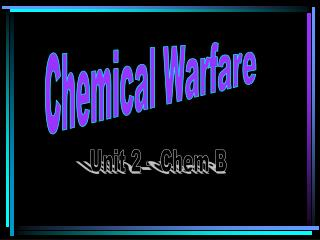DownloadDownload PresentationChemical Warfare

# Chemical Warfare

Télécharger la présentation## Chemical Warfare

- - - - - - - - - - - - - - - - - - - - - - - - - - - E N D - - - - - - - - - - - - - - - - - - - - - - - - - - -
##### Presentation Transcript

1. Chemical Warfare Unit 2 – Chem B

2. What is the balanced equation for the decomposition of hydrogen peroxide into water and oxygen gas? 2H2O2 2H2O + O2

3. What does the abbreviation STP stand for? Standard Temperature & Pressure

4. One mole of a compound contains how many particles? 6.02x1023 particles

5. 6.02x1023 is also known as Avagodro’s Number

6. The sum of the masses of the elements composing a compound is known as its Formula Mass

7. One mole of any gas has what molar volume? 22.4 Liters

8. The mass of each element in a compound divided by the formula mass is the Percent composition

9. What is the percent composition of CO2? %C = 12/44 = 27% %O = 16(2)/44 = 73%

10. Write the balanced formula for the reaction of iron with oxygen gas to form iron (III) oxide. 4Fe + 3O2 2Fe2O3

11. If 4.3g of iron reacts with oxygen, as in the previous formula, what mass of Fe2O3 would be produced? 6.1 g Fe2O3

12. How many liters of oxygen are required for the previous reaction? 1.29 L O2

13. Aluminum reacts with bromine gas to produce aluminum bromide. What is the balanced equation for this reaction? 2Al + 3Br2 2AlBr3

14. What type of reaction is the one previously written? 2Al + 3Br2 2AlBr3 Synthesis reaction

15. If 15.0g Al reacts with 46.0g Br2, which is the limiting reactant? 46.0g Br2 = 148.3g = 51.2g

16. Find the empirical formula for the compound that consists of 20.23% Al and 79.77% Cl. AlCl3 = 1 .75 = 3 .75

17. If the molar mass of the compound in the previous question is 264g, what is the molecular formula? Al2Cl6 AlCl3 formula mass = 132 264/132 = 2

18. For the formula 2P + 3Br2 2PBr3, if 5.0g P and 35g of Br2 react, what is the limiting reactant and how what mass of PBr3 is produced? 35g Br2 produces 39.5g PBr3 = 43.7g = 39.5g

19. If the actual yield of PBr3 from the previous problem is 30g, what is the percent yield? 75.9% 30g/39.5g

20. In the writing of an ionic compound, how do we determine the number of each atom in the compound? Criss cross the ionic charges

21. What law are we following when we balance a chemical equation? Law of Conservation of Mass

22. Balance the following equation: Ca(ClO3)2 CaCl2 + O2 Ca(ClO3)2 CaCl2 + 3O2

23. Write the balanced equation for the following word equation:Aluminum chloride + Calcium  Aluminum + Calcium chloride 2AlCl3 + 3Ca  2Al + 3CaCl2